• +91 9971497814
• info@interviewmaterial.com

# RD Chapter 27- Hyperbola Interview Questions Answers

### Related Subjects

Question 1 : The equation of the directrix of a hyperbola is x – y + 3 = 0. Its focus is (-1, 1) and eccentricity 3. Find the equation of the hyperbola.

Given:

The equation of the directrix of a hyperbola => x – y + 3= 0.

Focus = (-1, 1) and

Eccentricity = 3

Now, let us find the equation of the hyperbola

Let ‘M’ be the point on directrix and P(x, y) be any pointof the hyperbola.

By using the formula,

e = PF/PM

PF = ePM [where, e is eccentricity, PM is perpendicular fromany point P on hyperbola to the directrix]

So,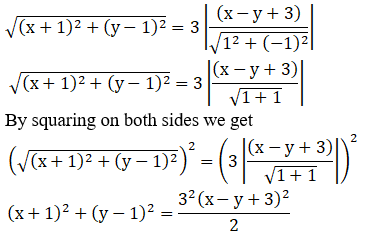[We know that (a – b)2 =a2 + b2 + 2ab &(a + b + c)2 =a2 + b2 + c2 + 2ab + 2bc + 2ac]

So, 2{x2 + 1 + 2x + y2 + 1 –2y} = 9{x2 + y2+ 9 + 6x – 6y – 2xy}

2x2 + 2 + 4x + 2y2 + 2 – 4y= 9x2 + 9y2+ 81 + 54x – 54y – 18xy

2x2 + 4 + 4x + 2y2– 4y – 9x2 –9y2 – 81 – 54x + 54y + 18xy = 0

– 7x2 – 7y2 – 50x + 50y +18xy – 77 = 0

7(x2 + y2) – 18xy + 50x – 50y +77 = 0

The equation of hyperbola is 7(x2 + y2)– 18xy + 50x – 50y + 77 = 0

Question 2 :
Find the equation of the hyperbola whose
(i) focus is (0, 3), directrix is x + y – 1 = 0 and eccentricity = 2
(ii) focus is (1, 1), directrix is 3x + 4y + 8 = 0 and eccentricity = 2
(iii) focus is (1, 1) directrix is 2x + y = 1 and eccentricity =√3
(iv) focus is (2, -1), directrix is 2x + 3y = 1 and eccentricity = 2
(v) focus is (a, 0), directrix is 2x + 3y = 1 and eccentricity = 2
(vi)focus is (2, 2), directrix is x + y = 9 and eccentricity = 2

(i) focus is (0, 3), directrix is x + y – 1 = 0 and eccentricity= 2

Given:

Focus = (0, 3)

Directrix => x + y – 1 = 0

Eccentricity = 2

Now, let us find the equation of the hyperbola

Let ‘M’ be the point on directrix and P(x, y) be any pointof the hyperbola.

By using the formula,

e = PF/PM

PF = ePM [where, e is eccentricity, PM is perpendicular fromany point P on hyperbola to the directrix]

So,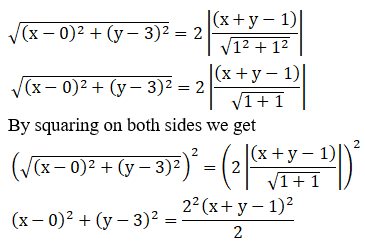[We know that (a – b)2 =a2 + b2 + 2ab &(a + b + c)2 =a2 + b2 + c2 + 2ab + 2bc + 2ac]

So, 2{x2 + y2 + 9 – 6y} =4{x2 + y2 + 1 – 2x – 2y + 2xy}

2x2 + 2y2 + 18 – 12y = 4x2 +4y2+ 4 – 8x – 8y + 8xy

2x2 + 2y2 + 18 – 12y – 4x2 –4y2 – 4 – 8x + 8y – 8xy = 0

– 2x2 – 2y2 – 8x – 4y – 8xy+ 14 = 0

-2(x2 + y2 – 4x + 2y + 4xy –7) = 0

x2 + y2 – 4x + 2y + 4xy – 7= 0

The equation of hyperbola is x2 + y2 –4x + 2y + 4xy – 7 = 0

(ii) focus is (1, 1), directrix is 3x + 4y + 8 = 0 andeccentricity = 2

Focus = (1, 1)

Directrix => 3x + 4y + 8 = 0

Eccentricity = 2

Now, let us find the equation of the hyperbola

Let ‘M’ be the point on directrix and P(x, y) be any pointof the hyperbola.

By using the formula,

e = PF/PM

PF = ePM [where, e is eccentricity, PM is perpendicular fromany point P on hyperbola to the directrix]

So,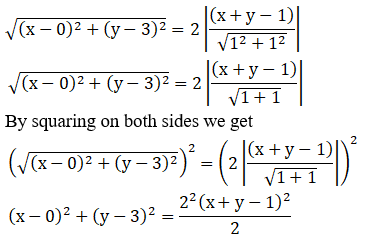[We know that (a – b)2 =a2 + b2 + 2ab &(a + b + c)2 =a2 + b2 + c2 + 2ab + 2bc + 2ac]

25{x2 + 1 – 2x + y2 + 1 –2y} = 4{9x2 + 16y2+ 64 + 24xy + 64y + 48x}

25x2 + 25 – 50x + 25y2 + 25– 50y = 36x2 + 64y2 + 256 + 96xy + 256y + 192x

25x2 + 25 – 50x + 25y2 + 25– 50y – 36x2 – 64y2 – 256 – 96xy – 256y –192x = 0

– 11x2 – 39y2 – 242x – 306y– 96xy – 206 = 0

11x2 + 96xy + 39y2 + 242x +306y + 206 = 0

The equation of hyperbola is11x2 + 96xy +39y2 + 242x + 306y + 206 = 0

(iii) focus is (1, 1) directrix is 2x + y = 1 and eccentricity =√3

Given:

Focus = (1, 1)

Directrix => 2x + y = 1

Eccentricity =√3

Now, let us find the equation of the hyperbola

Let ‘M’ be the point on directrix and P(x, y) be any pointof the hyperbola.

By using the formula,

e = PF/PM

PF = ePM [where, e is eccentricity, PM is perpendicular fromany point P on hyperbola to the directrix]

So,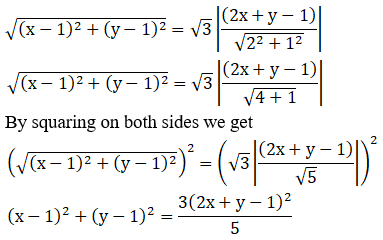[We know that (a – b)2 =a2 + b2 + 2ab &(a + b + c)2 =a2 + b2 + c2 + 2ab + 2bc + 2ac]

5{x2 + 1 – 2x + y2 + 1 – 2y}= 3{4x2 + y2+ 1 + 4xy – 2y – 4x}

5x2 + 5 – 10x + 5y2 + 5 –10y = 12x2 + 3y2 + 3 + 12xy – 6y – 12x

5x2 + 5 – 10x + 5y2 + 5 –10y – 12x2 – 3y2 – 3 – 12xy + 6y + 12x = 0

– 7x2 + 2y2 + 2x – 4y – 12xy+ 7 = 0

7x2 + 12xy – 2y2 – 2x + 4y–7 = 0

The equation of hyperbola is7x2 + 12xy – 2y2 –2x + 4y– 7 = 0

(iv) focus is (2, -1), directrix is 2x + 3y = 1 and eccentricity= 2

Given:

Focus = (2, -1)

Directrix => 2x + 3y = 1

Eccentricity = 2

Now, let us find the equation of the hyperbola

Let ‘M’ be the point on directrix and P(x, y) be any pointof the hyperbola.

By using the formula,

e = PF/PM

PF = ePM [where, e is eccentricity, PM is perpendicular fromany point P on hyperbola to the directrix]

So,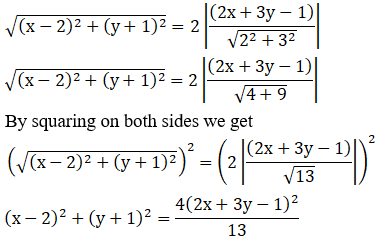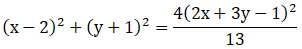[We know that (a – b)2 =a2 + b2 + 2ab &(a + b + c)2 =a2 + b2 + c2 + 2ab + 2bc + 2ac]

13{x2 + 4 – 4x + y2 + 1 +2y} = 4{4x2 + 9y2 + 1 + 12xy – 6y – 4x}

13x2 + 52 – 52x + 13y2 + 13+ 26y = 16x2 + 36y2 + 4 + 48xy – 24y – 16x

13x2 + 52 – 52x + 13y2 + 13+ 26y – 16x2 – 36y2 – 4 – 48xy + 24y +16x = 0

– 3x2 – 23y2 – 36x + 50y –48xy + 61 = 0

3x2 + 23y2 + 48xy + 36x –50y– 61 = 0

The equation of hyperbola is3x2 + 23y2 +48xy + 36x – 50y– 61 = 0

(v) focus is (a, 0), directrix is 2x + 3y = 1 and eccentricity =2

Given:

Focus = (a, 0)

Directrix => 2x + 3y = 1

Eccentricity = 2

Now, let us find the equation of the hyperbola

Let ‘M’ be the point on directrix and P(x, y) be any pointof the hyperbola.

By using the formula,

e = PF/PM

PF = ePM [where, e is eccentricity, PM is perpendicular fromany point P on hyperbola to the directrix]

So,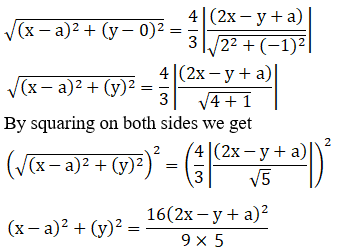[We know that (a – b)2 =a2 + b2 + 2ab &(a + b + c)2 =a2 + b2 + c2 + 2ab + 2bc + 2ac]

45{x2 + a2 – 2ax + y2}= 16{4x2 + y2 + a2 – 4xy – 2ay+ 4ax}

45x2 + 45a2 – 90ax + 45y2 =64x2 + 16y2 + 16a2 – 64xy –32ay + 64ax

45x2 + 45a2 – 90ax + 45y2 –64x2 – 16y2 – 16a2 + 64xy +32ay – 64ax = 0

19x2 – 29y2 + 154ax – 32ay –64xy – 29a2 = 0

The equation of hyperbola is19x2 – 29y2 +154ax – 32ay – 64xy – 29a2 = 0

(vi) focus is (2, 2), directrix is x + y = 9 and eccentricity = 2

Given:

Focus = (2, 2)

Directrix => x + y = 9

Eccentricity = 2

Now, let us find the equation of the hyperbola

Let ‘M’ be the point on directrix and P(x, y) be any pointof the hyperbola.

By using the formula,

e = PF/PM

PF = ePM [where, e is eccentricity, PM is perpendicular fromany point P on hyperbola to the directrix]

So,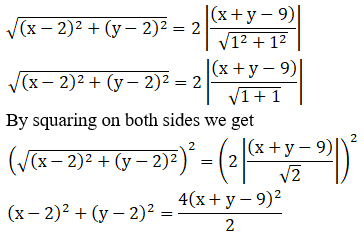[We know that (a – b)2 =a2 + b2 + 2ab &(a + b + c)2 =a2 + b2 + c2 + 2ab + 2bc + 2ac]

x2 + 4 – 4x + y2 + 4 – 4y =2{x2 + y2 + 81 + 2xy – 18y – 18x}

x2 – 4x + y2 + 8 – 4y = 2x2 +2y2 + 162 + 4xy – 36y – 36x

x2 – 4x + y2 + 8 – 4y – 2x2 –2y2 – 162 – 4xy + 36y + 36x = 0

– x2 – y2 + 32x + 32y + 4xy– 154 = 0

x2 + 4xy + y2 – 32x – 32y +154 = 0

The equation of hyperbola isx2 + 4xy + y2 –32x – 32y + 154 = 0

Question 3 :

Find the eccentricity, coordinatesof the foci, equations of directrices and length of the latus-rectum of thehyperbola.
(i) 9x2 – 16y2 = 144

(ii) 16x2 – 9y2 =-144

(iii) 4x2 – 3y2 =36

(iv) 3x2 – y2 =4

(v) 2x2 – 3y2 =5

(i) 9x2 – 16y2 = 144

Given:

The equation => 9x2 – 16y2 =144

The equation can be expressed as: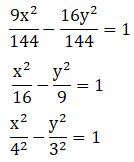The obtained equation is of the form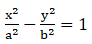Where, a2 = 16, b2 = 9 i.e.,a = 4 and b = 3

Eccentricity is given by: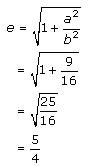Foci: The coordinates of the foci are (0, ±be)

(0, ±be) = (0, ±4(5/4))

= (0, ±5)

The equation of directrices is given as: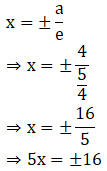5x 16 = 0

The length of latus-rectum is given as:

2b2/a

= 2(9)/4

= 9/2

(ii) 16x2 – 9y2 = -144

Given:

The equation => 16x2 – 9y2 =-144

The equation can be expressed as: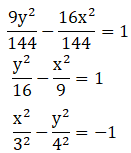The obtained equation is of the formWhere, a2 = 9, b2 = 16 i.e.,a = 3 and b = 4

Eccentricity is given by: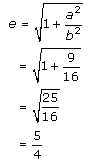Foci: The coordinates of the foci are (0, ±be)

(0, ±be) = (0, ±4(5/4))

= (0, ±5)

The equation of directrices is given as: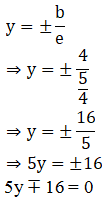The length of latus-rectum is given as:

2a2/b

= 2(9)/4

= 9/2

(iii) 4x2 – 3y2 = 36

Given:

The equation => 4x2 – 3y2 =36

The equation can be expressed as: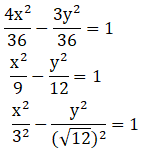The obtained equation is of the formWhere, a2 = 9, b2 = 12 i.e.,a = 3 and b = √12

Eccentricity is given by: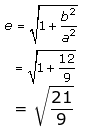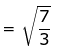Foci: The coordinates of the foci are (±ae, 0)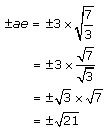(±ae, 0) = (±√21,0)

The equation of directrices is given as: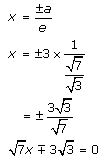The length of latus-rectum is given as:

2b2/a

= 2(12)/3

= 24/3

= 8

(iv) 3x2 – y2 = 4

Given:

The equation => 3x2 – y2 =4

The equation can be expressed as: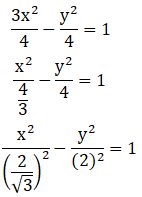The obtained equation is of the formWhere, a = 2/√3 and b = 2

Eccentricity is given by: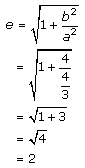Foci: The coordinates of the foci are (±ae, 0)

(±ae, 0) = ±(2/√3)(2) = ±4/√3

(±ae, 0) = (±4/√3, 0)

The equation of directrices is given as: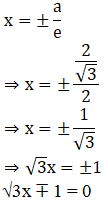The length of latus-rectum is given as:

2b2/a

= 2(4)/[2/√3]

= 4√3

(v) 2x2 – 3y2 = 5

Given:

The equation => 2x2 – 3y2 =5

The equation can be expressed as: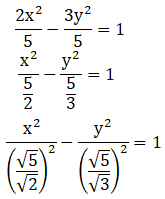The obtained equation is of the formWhere, a = √5/√2 and b = √5/√3

Eccentricity is given by: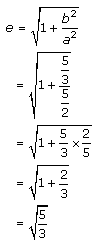Foci: The coordinates of the foci are (±ae, 0)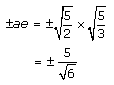(±ae, 0) = (±5/√6, 0)

The equation of directrices is given as: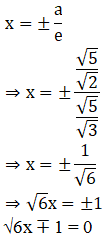The length of latus-rectum is given as:

2b2/a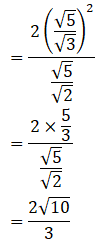Question 4 :

Find the axes, eccentricity,latus-rectum and the coordinates of the foci of the hyperbola 25x2 –36y2 = 225.

Given:

The equation=> 25x2 – 36y2 =225

The equation can be expressed as: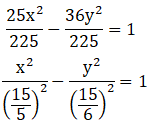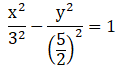The obtained equation is of the formWhere, a = 3 and b = 5/2

Eccentricity is given by: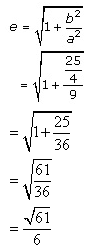Foci: The coordinates of the foci are (±ae, 0)

(±ae, 0) = ±3 (√61/6) = ± √61/2

(±ae, 0) = (± √61/2, 0)

The equation of directrices is given as: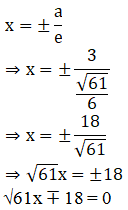The length of latus-rectum is given as:

2b2/a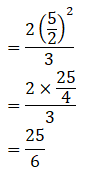Transverse axis = 6, conjugate axis = 5, e = √61/6, LR =25/6, foci = (± √61/2, 0)

Question 5 :

Find the centre, eccentricity,foci and directions of the hyperbola
(i) 16x2 – 9y2 + 32x + 36y – 164 = 0

(ii) x2 – y2 +4x = 0

(iii) x2 – 3y2 –2x = 8

(i) 16x2 – 9y2 + 32x + 36y – 164= 0

Given:

The equation => 16x2 – 9y2 +32x + 36y – 164 = 0

Let us find the centre, eccentricity, foci and directions ofthe hyperbola

By using the given equation

16x2 – 9y2 + 32x + 36y – 164= 0

16x2 + 32x + 16 – 9y2 + 36y– 36 – 16 + 36 – 164 = 0

16(x2 + 2x + 1) – 9(y2 – 4y+ 4) – 16 + 36 – 164 = 0

16(x2 + 2x + 1) – 9(y2 – 4y+ 4) – 144 = 0

16(x + 1)2 – 9(y – 2)2 = 144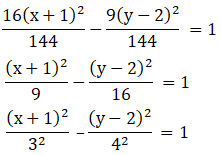Here, center of the hyperbola is (-1, 2)

So, let x + 1 = X and y – 2 = Y

The obtained equation is of the formWhere, a = 3 and b = 4

Eccentricity is given by: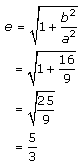Foci: The coordinates of the foci are (±ae, 0)

X = ±5 and Y = 0

x + 1 = ±5 and y – 2 = 0

x = ±5 – 1 and y = 2

x = 4, -6 and y = 2

So, Foci: (4, 2) (-6, 2)

Equation of directrix are: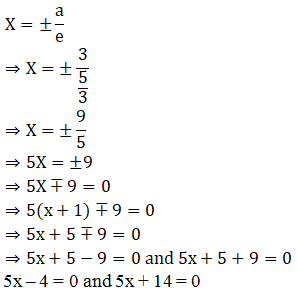The center is (-1, 2), eccentricity (e) = 5/3, Foci = (4,2) (-6, 2), Equation of directrix = 5x – 4 = 0 and 5x + 14 = 0

(ii) x2 – y2 + 4x = 0

Given:

The equation => x2 – y2 +4x = 0

Let us find the centre, eccentricity, foci and directions ofthe hyperbola

By using the given equation

x2 – y2 + 4x = 0

x2 + 4x + 4 – y2 – 4 = 0

(x + 2)2 – y2 = 4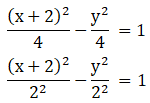Here, center of the hyperbola is (2, 0)

So, let x – 2 = X

The obtained equation is of the formWhere, a = 2 and b = 2

Eccentricity is given by: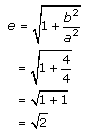Foci: The coordinates of the foci are (±ae, 0)

X = ± 2√2and Y = 0

X + 2 = ± 2√2and Y = 0

X= ± 2√2– 2 and Y = 0

So, Foci = (± 2√2– 2, 0)

Equation of directrix are: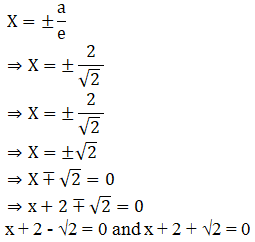The center is (-2, 0), eccentricity (e) = √2, Foci = (-2± 2√2, 0), Equation of directrix=

x + 2 = ±√2

(iii) x2 – 3y2 – 2x = 8

Given:

The equation => x2 – 3y2 –2x = 8

Let us find the centre, eccentricity, foci and directions ofthe hyperbola

By using the given equation

x2 – 3y2 – 2x = 8

x2 – 2x + 1 – 3y2 – 1 = 8

(x – 1)2 – 3y2 = 9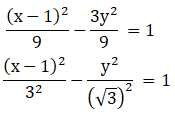Here, center of the hyperbola is (1, 0)

So, let x – 1 = X

The obtained equation is of the formWhere, a = 3 and b = √3

Eccentricity is given by: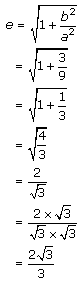Foci: The coordinates of the foci are (±ae, 0)

X = ± 2√3and Y = 0

X – 1 = ± 2√3and Y = 0

X= ± 2√3+ 1 and Y = 0

So, Foci = (1 ± 2√3,0)

Equation of directrix are: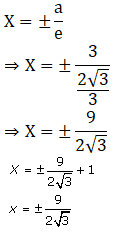The center is (1, 0), eccentricity (e) = 2√3/3, Foci =(1 ± 2√3, 0), Equationof directrix =

X = 1±9/2√3

Question 6 :
Find the equation of the hyperbola, referred to its principal axes as axes of coordinates, in the following cases:
(i) the distance between the foci = 16 and eccentricity = √2
(ii) conjugate axis is 5 and the distance between foci = 13
(iii) conjugate axis is 7 and passes through the point (3, -2)

(i) the distance between the foci = 16 and eccentricity =√2

Given:

Distance between the foci = 16

Eccentricity = √2

Let us compare with the equation of the formDistance between the foci is 2ae and b2 = a2(e2 –1)

So,

2ae = 16

ae = 16/2

a√2 = 8

a = 8/√2

a2 = 64/2

= 32

We know that, b2 = a2(e2 –1)

So, b2 = 32 [(√2)2 – 1]

= 32 (2 – 1)

= 32

The Equation of hyperbola is given as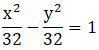x2 – y2 = 32

The Equation of hyperbola is x2 – y2 =32

(ii) conjugate axis is 5 and the distance between foci = 13

Given:

Conjugate axis = 5

Distance between foci = 13

Let us compare with the equation of the formDistance between the foci is 2ae and b2 = a2(e2 –1)

Length of conjugate axis is 2b

So,

2b = 5

b = 5/2

b2 = 25/4

We know that, 2ae = 13

ae = 13/2

a2e2 = 169/4

b2 = a2(e2 – 1)

b2 = a2e2 – a2

25/4 = 169/4 – a2

a2 = 169/4 – 25/4

= 144/4

= 36

The Equation of hyperbola is given as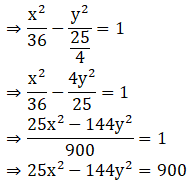The Equation of hyperbola is 25x2 – 144y2 =900

(iii) conjugate axis is 7 and passes through the point (3,-2)

Given:

Conjugate axis = 7

Passes through the point (3, -2)

Conjugate axis is 2b

So,

2b = 7

b = 7/2

b2 = 49/4

The Equation of hyperbola is given asSince it passes through points (3, -2)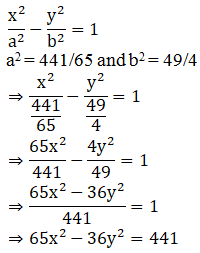The Equation of hyperbola is 65x2 – 36y2 =441

Todays Deals### RD Chapter 27- Hyperbola Contributorskrishan

Name:
Email:

# Latest News# 9000 interview questions in different categories